Next: Ionospheric Radio Wave Propagation Up: Wave Propagation in Inhomogeneous Previous: Total Internal Reflection

# Reflection by Conducting Surfaces

Suppose that the region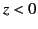is a vacuum, and the region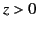is occupied by a good conductor of conductivity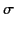. Consider a linearly polarized plane wave normally incident on the interface. Let the wave electric and magnetic fields in the vacuum region take the form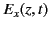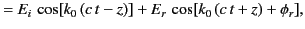(1042)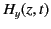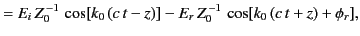(1043)

where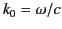is the vacuum wavenumber. Here,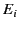and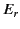are the amplitudes of the incident and reflected waves, respectively, whereas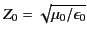. The wave electric and magnetic fields in the conductor are written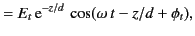(1044)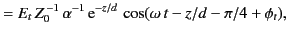(1045)

where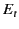is the amplitude of the evanescent wave that penetrates into the conductor,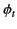is the phase of this wave with respect to the incident wave, and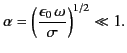(1046)

The appropriate matching conditions are the continuity of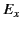and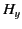at the vacuum/conductor interface (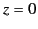). In other words,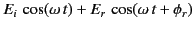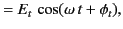(1047)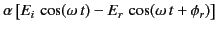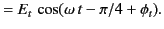(1048)

Equations (1049) and (1050), which must be satisfied at all times, can be solved, in the limit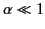, to give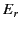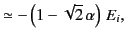(1049)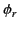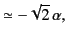(1050)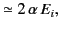(1051)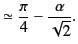(1052)

Hence, the coefficient of reflection becomes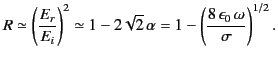(1053)

According to the previous analysis, a good conductor reflects a normally incident electromagnetic wave with a phase shift of almost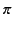radians (i.e.,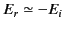). The coefficient of reflection is just less than unity, indicating that, while most of the incident energy is reflected by the conductor, a small fraction of it is absorbed.

High quality metallic mirrors are generally coated in silver, whose conductivity is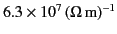. It follows, from Equation (1055), that at optical frequencies (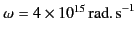) the coefficient of reflection of a silvered mirror is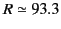percent. This implies that about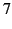percent of the light incident on the mirror is absorbed, rather than being reflected. This rather severe light loss can be problematic in instruments, such as astronomical telescopes, that are used to view faint objects.Next: Ionospheric Radio Wave Propagation Up: Wave Propagation in Inhomogeneous Previous: Total Internal Reflection
Richard Fitzpatrick 2014-06-27